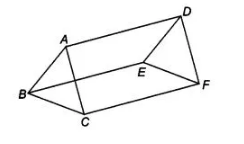# In figure, AB || DE, AB = DE, AC|| DF`
Question:

In figure, $A B\|D E, A B=D E, A C\| D F$ and $A C=O F$. Prove that $B C \| E F$ and $B C=E F$.Solution:

Given In figure AB || DE and AC || DF, also AB = DE and AC = DF

To prove BC ||EF and BC = EF

Proof In quadrilateral ABED, AB||DE and AB = DE

So, ABED is a parallelogram. AD || BE and AD = BE

Now, in quadrilateral ACFD, AC || FD and AC = FD …..(i)

Thus, ACFD is a parallelogram.

From Eqs. (i) and (ii), AD = BE = CF and CF || BE …(iii)

Now, in quadrilateral BCFE, BE = CF

and BE||CF [from Eq. (iii)]

So, BCFE is a parallelogram. BC = EF and BC|| EF . Hence proved.